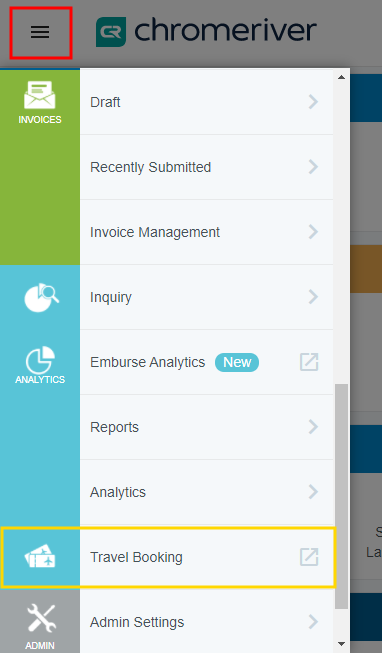Chrome River offers tight integration between Chrome River EXPENSE and such travel solution providers as Sabre, Amadeus, Egencia and Deem. Your organization may request to have a Travel Booking link added to the menu bar to take users directly to your travel partner.

The link is configurable via security rules, allowing you to determine which groups of users may see it and where it takes them. For example, if your organization uses a different travel partner in another country, the link can be configured based on the location in users' profiles. Note that delegates are not able to see the Travel Booking link when working for users who have access to it.

If you are interested in taking advantage of this feature, contact us via the Chrome River Help Desk.<% if (allItems.length > 1) { %>

<% } %>
<% var getColumnClasses = function(numberColumns) { var classNames = 'col-12'; if (numberColumns >= 2) classNames += ' md:col-6'; if (numberColumns >= 3) classNames += ' lg:col-4'; if (numberColumns >= 4) classNames += ' xl:col-3'; return classNames; } %>

##<%= heading %>

<% } %>
<% var getColumnClasses = function(numberColumns) { var classNames = 'col-12'; if (numberColumns >= 2) classNames += ' md:col-6'; if (numberColumns >= 3) classNames += ' lg:col-4'; if (numberColumns >= 4) classNames += ' xl:col-3'; return classNames; } %>

##<%= heading %>

<% } %>
<% var maxSections = 5 %>
<% if (sections.length) { %>
<% sections.forEach(function(section) { %>
• <%= partial('partial-article-list-sections', { id: 'section-' + section.id, parentId: '#' + id, sections: section.sections, activeCategoryId: activeCategoryId, activeSectionId: activeSectionId, activeArticleId: activeArticleId, partial: partial }) %> <% if (section.articles.length) { %> <% } %>
• <% }); %>
<% } %>
<% if (sections.length) { %>
<% sections.slice(0, maxSections).forEach(function(section) { %>
• <%= section.name %> <%= partial('partial-section-list-sections', { parent: section, sections: section.sections, maxSections: maxSections, partial: partial }) %>
• <% }); %> <% if (sections.length > maxSections) { %>
• See more
• <% } %>
<% } %>
<% var getColumnClasses = function(numberColumns) { var classNames = numberColumns === 'auto' ? 'col-auto' : 'col-12'; if (numberColumns >= 2) classNames += ' md:col-6'; if (numberColumns >= 3) classNames += ' lg:col-4'; if (numberColumns >= 4) classNames += ' xl:col-3'; return classNames; } %>
<% blocks.forEach(function(block, index) { %>
• <% if (imageHeight) { %><% } %>
<% if (block.name) { %>

<% if (block.html_url) { %> <%= block.name %> <% } else { %> <%= block.name %> <% } %>

<% } %> <% if (block.description) { %>

<%= block.description %>

<% } %>
• <% }) %>